Next: Two versions of QRA Up: Probabilistic Encryption Previous: The magic of sampling   Contents

## Determining algorithm performance by sampling

We are interested in algorithms for deciding whether a given number is or is not a square. As with the algorithm in Section 6, there is some probability that, for a given input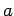, the algorithm may give the wrong answer.

Letbe the probability that a given algorithm gives the correct answer for input. We are also interested in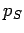, which is the average ofover all squares, and, the average over all pseudo-squares, and, the average ofover all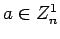.

If we are given an algorithm, we can easily determineby running it with inputon a sample of randomly chosen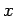and counting the number of times the algorithm answers this is a square.''

The procedure for determiningis more elaborate. Suppose we have an algorithm for which. Using Lemma 26, generate a sample of 100 members of, and run the algorithm on each of them. Suppose we get the answer this is a square'' 65 times. There aresquares in the sample, on which there have been .6(50) correct responses and 20 incorrect. Pseudo-squares have been identified as squares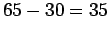times, which suggests. Finally.

Lemma 24 or 25 can be used to determine the probability that these estimates come within a specified amount.Next: Two versions of QRA Up: Probabilistic Encryption Previous: The magic of sampling   Contents
Translated from LaTeX by Scott Sutherland
2002-12-14